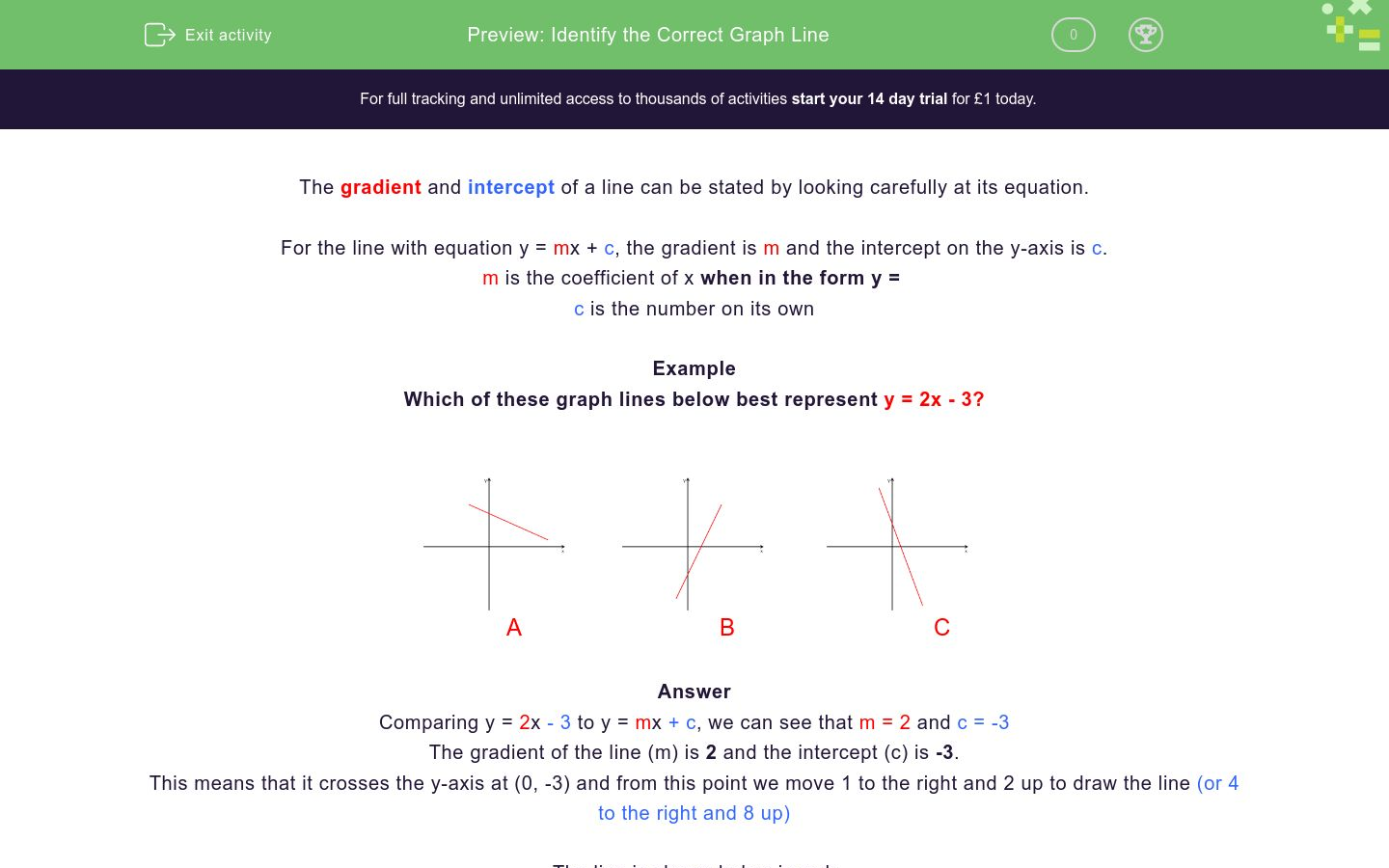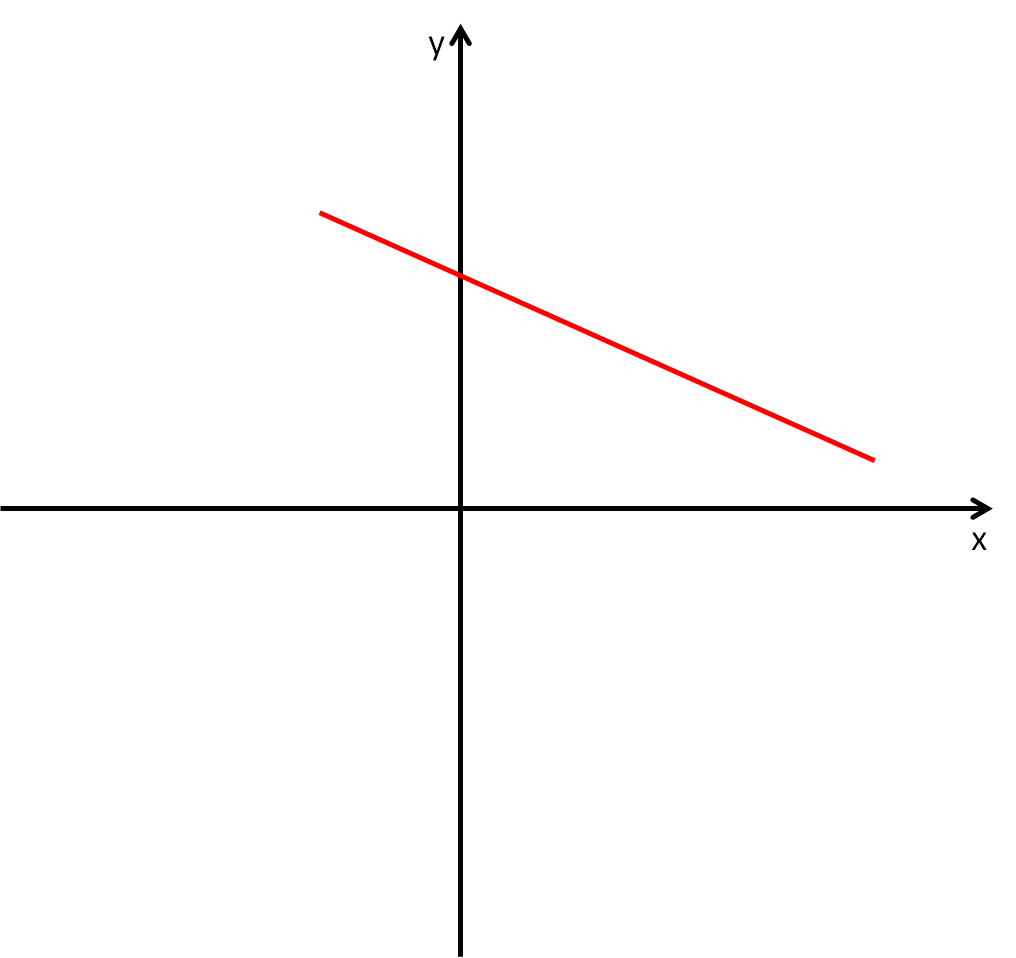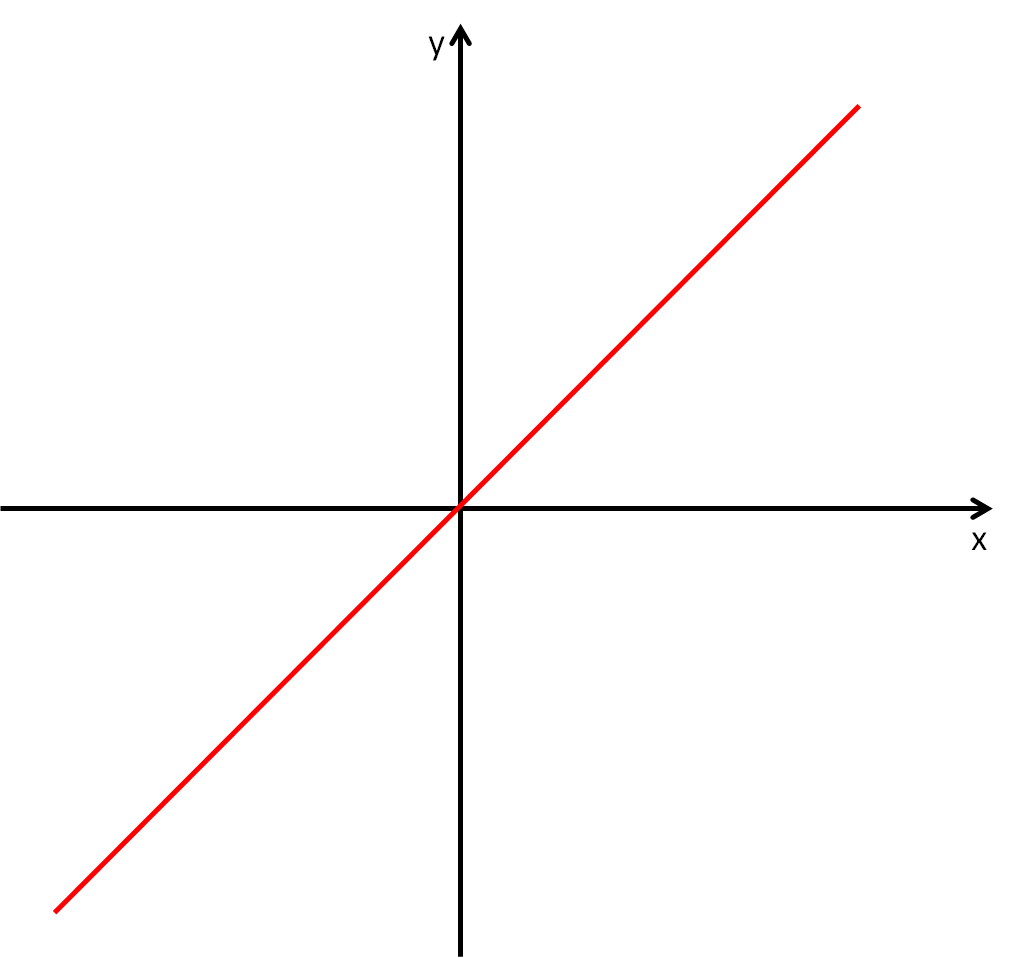# Choose the Graph Line

In this worksheet, students select the graph line that matches the given equation.Key stage:  KS 4

Curriculum topic:  Algebra

Difficulty level:### QUESTION 1 of 10

The gradient and intercept of a line can easily be stated by looking carefully at its equation.

For the line with equation y = mx + c, the gradient is m and the intercept on

the y-axis is c.

m is the coefficient of x when in the form y = ......

c is the number on its own

Example

Which of these graph lines best represents y = 2x - 3 ?A                            B                            C

Comparing y = 2- 3 to y = mx + c, we see that m = 2 and c = -3

The gradient of the line, m, is 2 and the intercept, c, is -3.

This means that it crosses the y-axis at (0, -3) and from this point we move 1 to the right and 2 up to draw the line (or 4 to the right and 8 up)

The line is shown below in red.So B is the only possible graph line that looks like this.  It has a positive gradient and a negative intercept on the y-axis.

NB  Assume that the scales on each axis are the same for all questions in this worksheet.

Which of these graph lines best represents y = 2x - 4 ?A                            B                            C

A

B

C

Which of these graph lines best represents y = 4 - 2x ?A                            B                            C

A

B

C

Which of these graph lines best represents y = 5 - 3x ?A                            B                            C

A

B

C

Which of these graph lines best represents y = 4 - ½x ?A                            B                            C

A

B

C

Which of these graph lines best represents y = 4 ?A                            B                            C

A

B

C

Which of these graph lines best represents x = 6 ?A                            B                            C

A

B

C

Which of these graph lines best represents y = -x ?A                            B                            C

A

B

C

Which of these graph lines best represents x = y ?A                            B                            C

A

B

C

Which of these graph lines best represents x = -2 ?A                            B                            C

A

B

C

Which of these graph lines best represents y = -5 + 2x ?A                            B                            C

A

B

C

• Question 1

Which of these graph lines best represents y = 2x - 4 ?A                            B                            C

B
• Question 2

Which of these graph lines best represents y = 4 - 2x ?A                            B                            C

C
• Question 3

Which of these graph lines best represents y = 5 - 3x ?A                            B                            C

C
• Question 4

Which of these graph lines best represents y = 4 - ½x ?A                            B                            C

A
• Question 5

Which of these graph lines best represents y = 4 ?A                            B                            C

B
• Question 6

Which of these graph lines best represents x = 6 ?A                            B                            C

A
• Question 7

Which of these graph lines best represents y = -x ?A                            B                            C

B
• Question 8

Which of these graph lines best represents x = y ?A                            B                            C

C
• Question 9

Which of these graph lines best represents x = -2 ?A                            B                            C

B
• Question 10

Which of these graph lines best represents y = -5 + 2x ?A                            B                            C